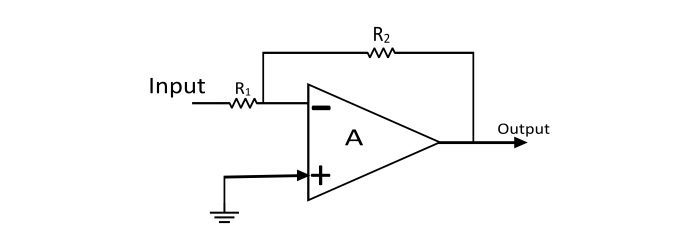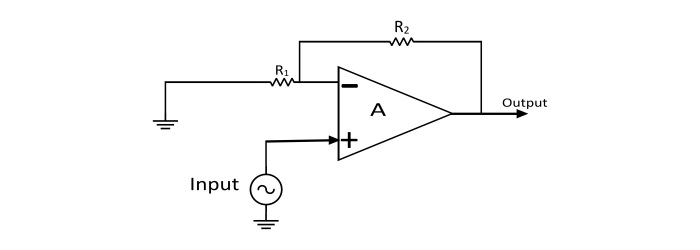# Inverting and Non-Inverting Operational Amplifiers

An operational amplifier is a three-terminal device consisting of two high impedance input terminals, one is called the inverting input denoted by a negative sign and the other is the non-inverting input denoted with a positive sign. The third terminal is the output of the Op-Amp.

## Inverting Operational Amplifier

In the inverting operational amplifier circuit, the signal is applied at the inverting input and the non-inverting input is connected to the ground. In this type of amplifier, the output is 180⁰ out of phase to the input, i.e. when positive signal is applied to circuit, the output of the circuit will be negative. By assuming the Op-Amp is ideal, then the concept of virtual short can be applied at the input terminals of the Op-Amp. So that voltage at the inverting terminal is equal to the voltage at non-inverting terminal.Applying KCL at inverting node of Op-Amp

$$\mathrm{\frac{0-V_{in}}{R_{1}}+\frac{0-V_{out}}{R_{2}}=0}$$

$$\mathrm{Voltage\:Gain(A_{v})=\frac{V_{out}}{V_{in}}=-\frac{R_{2}}{R_{1}}}$$

## Non-Inverting Operational Amplifier

When the signal is applied at the non-inverting input, the resulting circuit is known as Non-Inverting Op-Amp. In this amplifier the output is exactly in phase with the input i.e. when a positive voltage is applied to the circuit, the output will also be positive. By assuming the Op-Amp is ideal, then concept of virtual short can be applied i.e. the voltage at the inverting and non-inverting terminal is equal.Applying KCL at the inverting node

$$\mathrm{\frac{V_{in}-V_{out}}{R_{2}}+\frac{V_{0}-0}{R_{1}}=0}$$

$$\mathrm{Voltage\:Gain(A_{v})=\frac{V_{out}}{V_{in}}=1+\frac{R_{2}}{R_{1}}}$$

## Difference between Inverting and Non-Inverting Op-Amps

Inverting Op-AmpNon-Inverting Op-Amp
The type of feedback used is voltage shunt.The type of feedback used is voltage series.
The input and output voltages of this amplifier are 180⁰ out of phase.The input and output voltages are in phase.
$$\mathrm{Voltage\:Gain(A_{v})=\frac{V_{out}}{V_{in}}=-\frac{R_{2}}{R_{1}}}$$
$$\mathrm{Voltage\:Gain(A_{v})=\frac{V_{out}}{V_{in}}=1+\frac{R_{2}}{R_{1}}}$$
The input impedance is R1.The input impedance is very high.